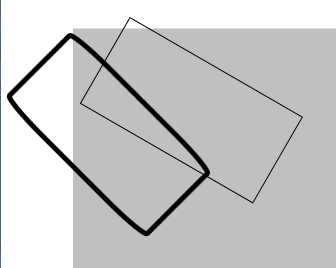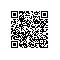# WPF快速指导14：变换

WPF快速指导14：变换

1：变换之RotateTransform
RotateTransform 就是抽象类Transform的一个子类，它负责在二维 x-y 坐标系内围绕指定点顺时针旋转对象。如，对一个rectangle转换角度45‘，前台代码如下：

<Rectangle.RenderTransform>
<RotateTransform Angle="45"></RotateTransform>
</Rectangle.RenderTransform>
</Rectangle>

<Rectangle x:Name="Rect2" Width="200" Height="100" Stroke="Black">
</Rectangle>

Rect2.RenderTransform = new RotateTransform() { Angle = 30 };2：变换之ScaleTransform

ScaleTransform也是抽象类Transform的一个子类，它负责在二维 x-y 坐标系内缩放对象。

<TextBlock x:Name="textBlock1" Background="Blue" Width="100" Height="50">abc</TextBlock>

textBlock1.RenderTransform = new ScaleTransform() { ScaleX = 1.5, ScaleY = 0.5 };

3：变化之TranslateTransform
TranslateTransform也是抽象类Transform的一个子类，它负责在二维 x-y 坐标系内平移（移动）对象。

<TextBlock x:Name="textBlock1" Background="Blue" Width="100" Height="50">abc</TextBlock>
<Button x:Name="buttonTest" Click="buttonTest_Click" Canvas.Top="250">测试</Button>

private void buttonTest_Click(object sender, RoutedEventArgs e)
{
textBlock1.RenderTransform
= new TranslateTransform() { X = 50, Y = 100 };
}

4：变化之SkewTransform
SkewTransform也是抽象类Transform的一个子类，它负责表示二维扭曲，可用于在二维对象中创建三维深度幻觉。

textBlock1.RenderTransform = new SkewTransform() { AngleX = 30, AngleY = 20, CenterX = 50, CenterY = 80 };

5：变化之MatrixTransform

6：变换合并

TransformGroup group = new TransformGroup();
new SkewTransform() { AngleX = 30, AngleY = 20, CenterX = 50, CenterY = 80 });
textBlock1.RenderTransform
= group;

private void buttonTest_Click(object sender, RoutedEventArgs e)
{
TransformGroup group
= ((textBlock1.RenderTransform) as TransformGroup);
new TranslateTransform() { X = 50, Y = 100 });
textBlock1.RenderTransform
= group;
}本文基于Creative Commons Attribution 2.5 China Mainland License发布，欢迎转载，演绎或用于商业目的，但是必须保留本文的署名http://www.cnblogs.com/luminji（包含链接）。如您有任何疑问或者授权方面的协商，请给我留言。使用钉钉扫一扫加入圈子
+ 订阅